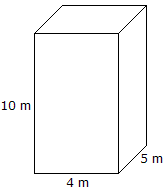# C Program Volume Of Cuboid | C Programs

C program to find the volume of a cuboid – In this stipulated article, we will talk about the multiple ways of how to find the volume of a cuboid in C programming.

Several suitable examples and sample outputs have been added as well. The compiler has been added for the proper understanding of the following C programming codes.

The multitude of methods used in this specific blog post to find the volume of a cuboid are as follows:

• Using Standard Method
• Using Functions
• Using Pointers
• Using Macros

A cuboid, as we all know, is a three-dimensional closed figure which resembles a rectangle from all of its six faces. A cuboid’s opposite sides are equal and parallel.

All of the angles are right angles in nature. This is how a cuboid looks like:As you can see, this is how a cuboid looks like. There are quite a few things in our daily life that resemble a cuboid like bricks, mobiles, folded laptops etc.

The volume of this particular cuboid can be calculated with this formula:

Volume = Length * Breadth * Height.

Hence, the volume of this cuboid is

Volume = 10 * 4 * 5 = 200 sq m.

Thus, the ways to calculate the volume of a cuboid in C programming are as follows:

## Using Standard Method

1)The formula for the volume of a cuboid is v=l*b*h, using this standard formula we are calculating volume.

2)Taking the length, breadth, height and substitute those values into variables “length, breadth, height”.

3)Calculated value will be stored into the variable “volume”

output:

### Using Functions

1)float volume(float l,float b,float h)

{return (i*b*h);} is the body of function.

2)We are calling the function using volume(l,b,h), the cursor goes to function body and calculate the volume and return the value.

3)the return value will store into the variable “v”.

output:

#### Using Pointers

1)*i,*b,*h,*v are pointer variables, float volume(float *l,float *b,float *h,float *v) function having the pointers as arguments.

2)we are calling the function using “volume(&l,&b,&h,&v)” by passing addresses of l,b,h,v

3)volume (float *l,float *b,float *h,float *v) function calculate the volume and return the value,that return value will stored into pointer variable *v.

output:
##### Using Macros

1)Here volume(l,b,h) is symbolic name  to l*b*h

2) volume(l,b,h)replaced with that expression given at #define.

output:
x

## C Program To Print Number Of Days In A Month | Java Tutoring

C program to input the month number and print the number of days in that ...